# Gel'fond-Schneider method

(Redirected from Gel'fond–Schneider method)
Jump to: navigation, search

In 1934 Hilbert's seventh problem (cf. also Hilbert problems) was solved independently by A.O. Gel'fond [a3] and Th. Schneider [a8]: Ifis a non-zero algebraic number,a non-zero logarithm ofandan irrational algebraic number, then the number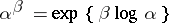is transcendental (cf. Transcendental number).

The transcendence of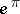(corresponding to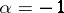,,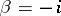) had already been proved by Gel'fond in 1929 [a2] using interpolation formulas for the function, like in Pólya's work [a7] on integral-valued entire functions.

One main common feature of both the Gel'fond and the Schneider method is to start with the construction of an auxiliary function by means of Dirichlet's box principle (the Thue–Siegel lemma; cf. also Dirichlet principle).

While Schneider's proof (cf. Schneider method) is based on the addition theorem for the exponential function, the main ingredient in Gel'fond's proof is the differential equation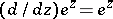. Gel'fond considers the two functionsand; his auxiliary function has the form, whereis a polynomial with algebraic coefficients. He investigates the values ofas well as its derivatives at the points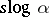,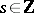. An extrapolation is an essential feature of his proof.

This method has been developed by Gel'fond himself for proving quantitative Diophantine approximation estimates (see [a4]; see also Gel'fond–Baker method; Diophantine approximations), and by Schneider, who obtained an extension of the Gel'fond–Schneider theorem to elliptic and Abelian functions: he proved the transcendence of elliptic integrals of the first or second kind [a9] and of Abelian integrals [a10], including the transcendence of the values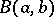of the beta-function at rational points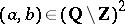. Next, Schneider [a11], [a12] provided general statements on the algebraic values of analytic functions satisfying differential equations; these results have been simplified and improved in the 1960s by S. Lang [a5], who extended Schneider's results to commutative algebraic groups. The following far-reaching statement is called the Schneider–Lang criterion: Letbe a number field and let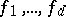be meromorphic functions inof finite order of growth (cf. also Meromorphic function). Assume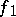,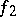are algebraically independent (cf. also Algebraic independence). Assume also that for, the derivative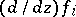of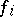belongs to the ring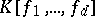. Then the set ofthat are not poles of anyand such that, for, is finite.

Schneider and Lang extended their criterion to several variables by considering Cartesian products; a deeper result, involving algebraic hypersurfaces and suggested by M. Nagata [a5], has been obtained by E. Bombieri [a1].

A clever modification of the Gel'fond–Schneider method has been applied to modular functions in [a13], solving Mahler's conjecture: For any algebraic numberwiththe valueof the modular function is transcendental.

Gel'fond proved in 1949 the algebraic independence ofand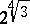. More generally, he proved that for algebraicandwith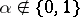andof degree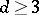, the transcendence degree overof the fieldis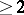(cf. also Transcendental extension). After the work of G.V. Chudnovskii, P. Philippon and G. Diaz, it is known that this transcendence degree is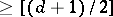. This method not only provides a new proof of the Lindemann–Weierstrass theorem on the algebraic independence of numbers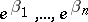when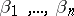are-linearly independent algebraic numbers, but also yields a similar result for elliptic functions (and, more generally, Abelian functions), as shown by Philippon and G. Wüstholz.

Also, Chudnovskii proved the algebraic independence of the two numbers,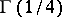(showing therefore thatis transcendental), and later Yu.V. Nesterenko adapted the method of [a13] and obtained remarkable results of algebraic independence on values of modular functions, including the algebraic independence of the three numbers,and[a6].

In another direction, both the Gel'fond and the Schneider method have been extended in order to prove results of linear independence over the field of algebraic numbers of logarithms of algebraic numbers (see Schneider method and Gel'fond–Baker method).